# User Guide: 3 Plotting transformed data

## Introduction

Package ggspectra extends ggplot2 with stats, geoms, scales and annotations suitable for light spectra. It also defines ggplot() and autoplot() methods specialized for the classes defined in package photobiology for storing different types of spectral data. The autoplot() methods are described separately in vignette User Guide: 2 Autoplot Methods and the ggplot() methods, statistics, and scales in User Guide: 1 Grammar of Graphics.

The new elements can be freely combined with methods and functions defined in packages ‘ggplot2’, scales, ggrepel, cowplot and other extensions to ‘ggplot2’.

This third part of the User Guide describes how to combine manipulation of spectral data with plotting. This streamlined coding is made possible by the enhancement implemented in ‘ggspectra’ (>= 0.3.5). In addition, some of the examples make use of methods available only in ‘photobiology’ (>= 0.10.0).

In ‘ggspectra’ (>= 0.3.5) the data member of gg (ggplot) objects remains as an object of the classes for spectral data defined in ‘photobiology’ instead of being converted into a plain data.frame. This makes it possible data manipulations in layers to be done with methods specific to spectral data.

The examples in this vignette depend conditionally on packages ‘rlang’ and ‘magrittr’. If these packages are not avialble when the vignette is built, the code chunks that require them are not evaluated.

## Set up

library(ggplot2)
library(photobiology)
library(photobiologyWavebands)
library(ggspectra)
# if suggested packages are available
magrittr_installed <- requireNamespace("magrittr", quietly = TRUE)
rlang_installed <- requireNamespace("rlang", quietly = TRUE)
eval_chunks <- magrittr_installed && rlang_installed
if (eval_chunks) {
library(magrittr)
library(rlang)
} else {
message("Please, install packages 'rlang' and 'magrittr'.")
}
##
## Attaching package: 'rlang'
## The following object is masked from 'package:magrittr':
##
##     set_names

Create a collection of two source_spct objects.

two_suns.mspct <- source_mspct(list(sun1 = sun.spct, sun2 = sun.spct / 2))

We bind the two spectra in the collection into a single spectral object. This object includes an indexing factor, by default named spct.idx. We use this new object to later on demonstrate grouping in ggplots.

two_suns.spct <- rbindspct(two_suns.mspct)

We change the default theme.

theme_set(theme_bw())

## Visualizing the effect of methods

The class of the spectral objects stored by calls to ggplot() methods specific to them is no longer stripped, neither are other attributes used by package ‘photobiology’. Consequently, transformations in layers using default data can use methods from package ‘photobiology’.

A simple example passing spectral data to each layer function follows. This approach was previously the only available.

ggplot() +
geom_line(data = sun.spct, mapping = aes(w.length, s.e.irrad)) +
geom_line(data = sun.spct %>% smooth_spct(method = "supsmu"),
colour = "red", size = 1.2)

The code can be simplified usinf . to refer to the default data in the ggplot object. This is possible because the argument passed to data in the call to ggplot2() is not converted into a “plain” data frame. In this case, it remains as an object of class source_spct. The mapping to aesthetics remains valid because smooth_spct() returns a new source_spct object with the same column names as sun.spct, which was passed as argument to data.

ggplot(sun.spct) +
geom_line() +
geom_line(data = . %>% smooth_spct(method = "supsmu"),
colour = "red", size = 1.2)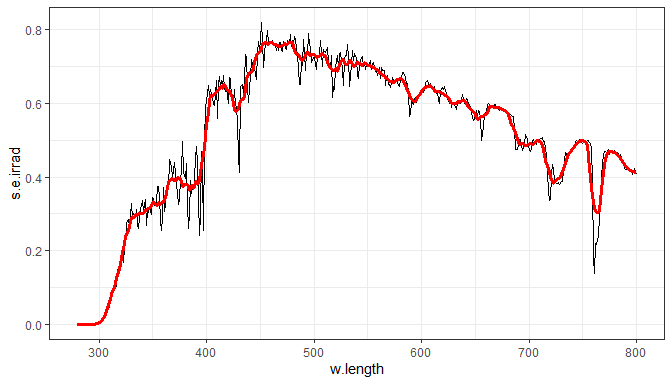The easiest approach to plotting photon spectral irradiance instead of spectral energy irradiance is to temporarily change the default radiation unit. An alternative approach is to replace the first two lines in the code chunk below by: ggplot(sun.spct, unit.out = "photon").)

photon_as_default()
ggplot(sun.spct) +
geom_line() +
geom_line(data = . %>% smooth_spct(method = "supsmu"),
colour = "red", size = 1.2)
unset_radiation_unit_default()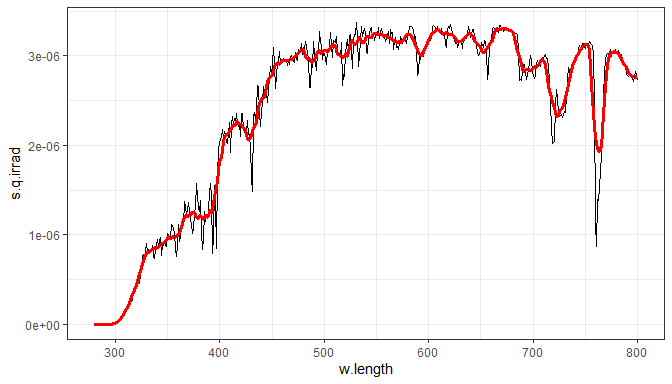Obviously, the default data does not need to be plotted, so this provides a roundabout way of applying methods,

ggplot(sun.spct) +
geom_line(data = . %>% smooth_spct(method = "supsmu"),
colour = "red", size = 1.2)

which is equivalent to doing the transformation ahead of plotting, which might be preferable.

sun.spct %>%
smooth_spct(method = "supsmu") %>%
ggplot() +
geom_line(colour = "red", size = 1.2)

However, when using different transformations in different layers we need to apply them at each layer.

ggplot(sun.spct) +
geom_line() +
geom_line(data = . %>% smooth_spct(method = "custom"),
colour = "blue", size = 1) +
geom_line(data = . %>% smooth_spct(method = "lowess"),
colour = "green", size = 1) +
geom_line(data = . %>% smooth_spct(method = "supsmu"),
colour = "red", size = 1)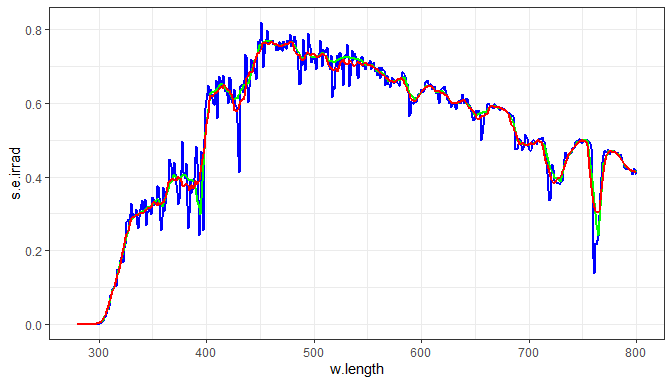Of course, this approach works both with geoms and stats, but one should remember that these layer functions do not “see” the original data object, but instead a new data frame containing the mapped variables in columns named according to aesthetics. The next example demostrates this and illustrates that smoothing displaces the wavelength of maximum spectral irradiance.

ggplot(sun.spct) +
geom_line() +
stat_peaks(size = 3, span = NULL) +
stat_peaks(geom = "vline", linetype = "dotted", span = NULL) +
geom_line(data = . %>% smooth_spct(method = "supsmu"),
colour = "red", size = 1.2) +
stat_peaks(data = . %>% smooth_spct(method = "supsmu"),
colour = "red", size = 3, span = NULL) +
stat_peaks(data = . %>% smooth_spct(method = "supsmu"),
geom = "vline", colour = "red",
linetype = "dotted", span = NULL)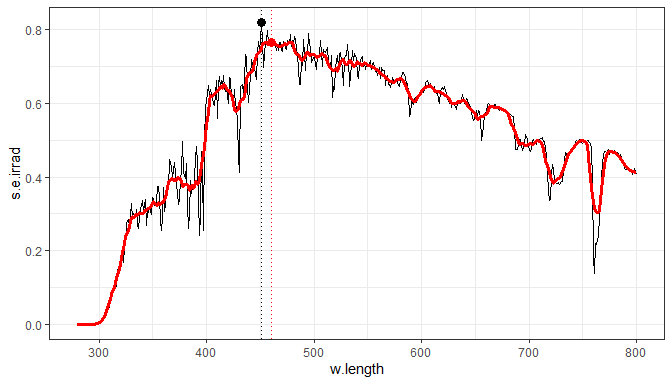We can easily highlight a wavelength range by overplotting the line in a different color.

ggplot(sun.spct) +
geom_line() +
geom_line(data = . %>% trim_wl(range = PAR()), colour = "red")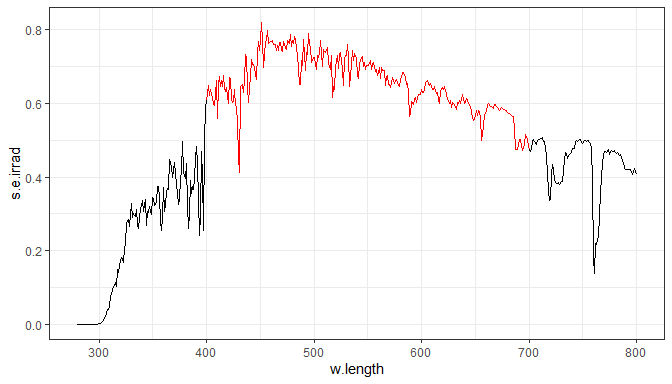We can highlight a range of wavelengths by plotting the points using colors matching human color vision.

ggplot(sun.spct) +
geom_line() +
geom_point(data = . %>% trim_wl(range = VIS()) %>% tag(),
mapping = aes(color = wl.color),
shape = "circle", size = 1.3) +
scale_color_identity()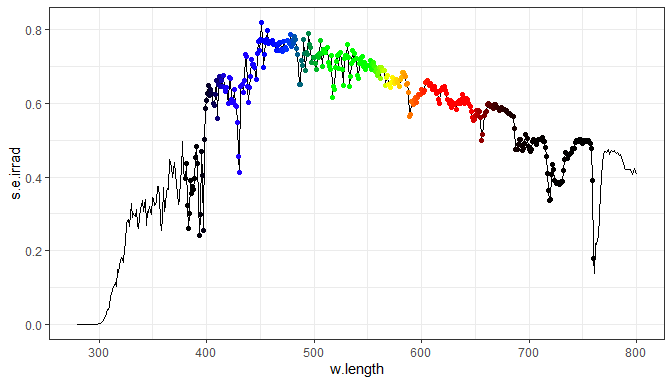In the plot above, spectral irradiance is taken into account when computing the colors, while below, only the wavelength at the centre of each waveband is used.

ggplot(sun.spct) +
geom_area(data = . %>% trim_wl(range = VIS()) %>% tag(w.band = VIS_bands()),
mapping = aes(fill = wb.color)) +
geom_line() +
scale_fill_identity()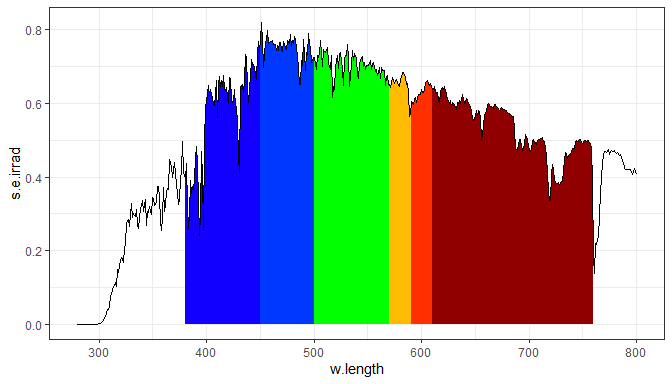Some of the methods from ‘photobiology’ are also defined for data.frame and can be used as summary functions with data that are not radiation spectra, such as any data seen by layer functions including stat_summary(). Furthermore, on-the-fly summaries and transformation can be used in any ggplot layer function and with any suitable function acepting data frames as input.